# EM I Griffiths Chapter 2 Electrostatics Coulombs Law

• Slides: 64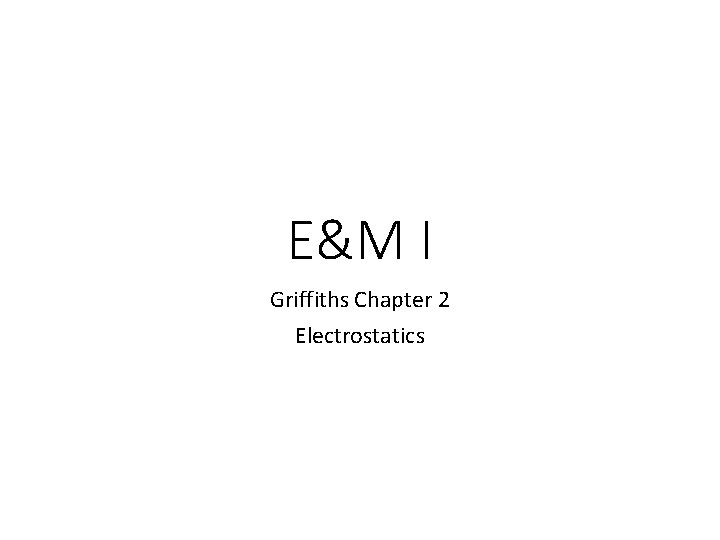E&M I Griffiths Chapter 2 Electrostatics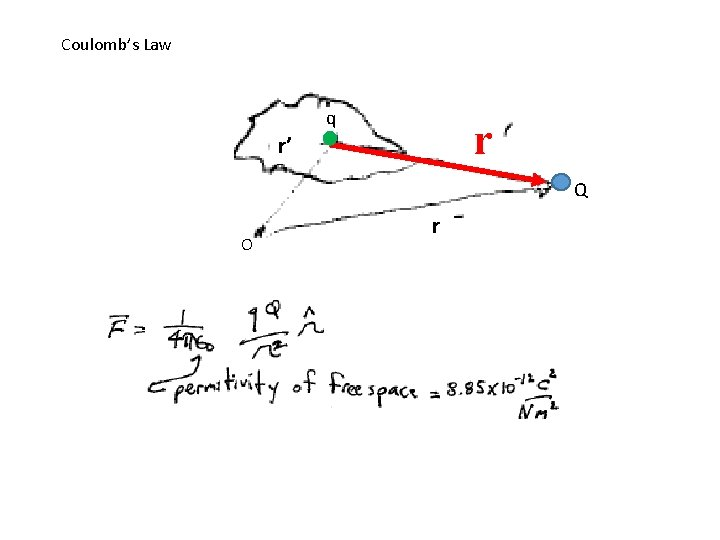Coulomb’s Law q r r’ Q O r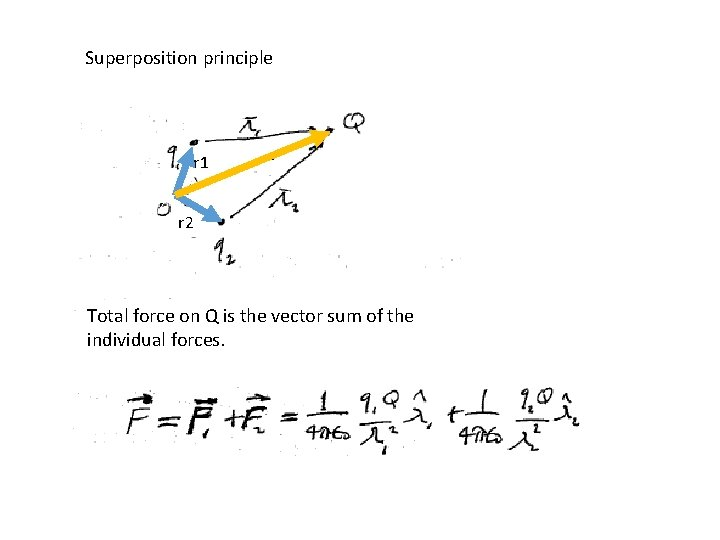Superposition principle r 1 r r 2 Total force on Q is the vector sum of the individual forces.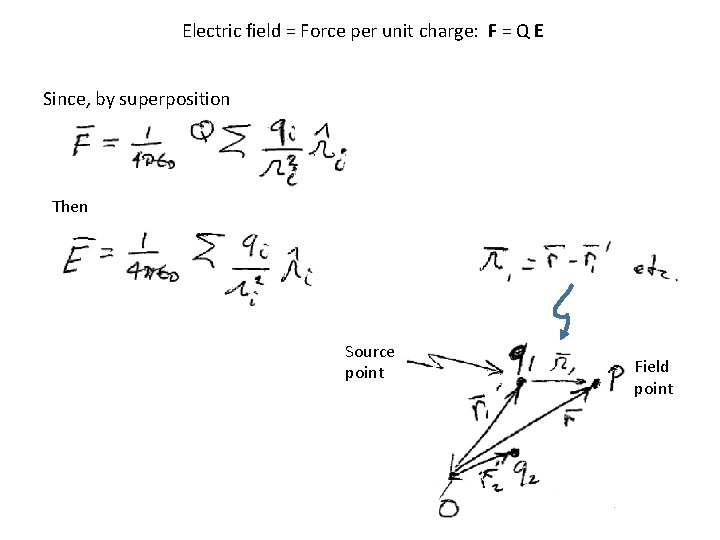Electric field = Force per unit charge: F = Q E Since, by superposition Then Source point Field point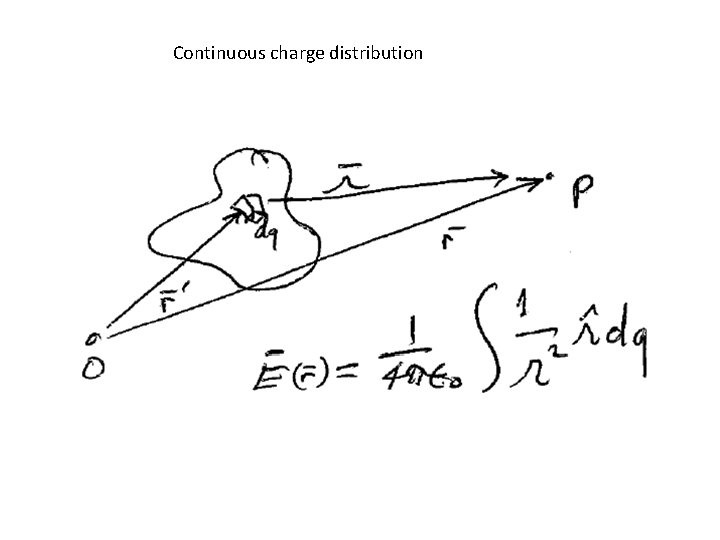Continuous charge distribution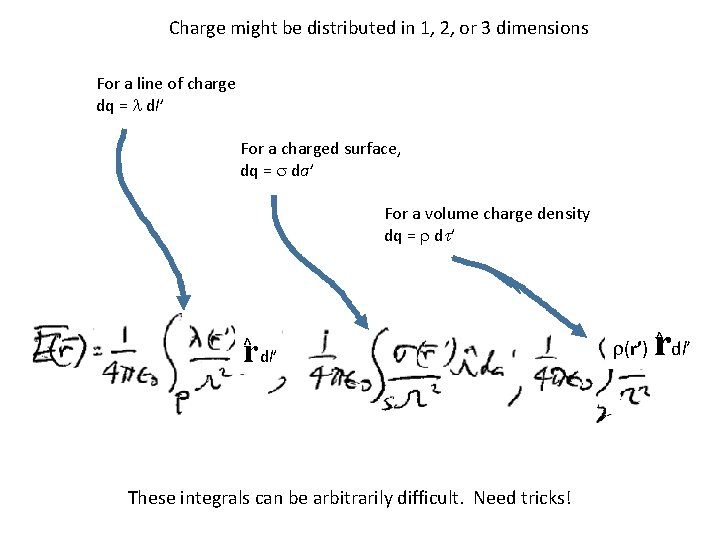Charge might be distributed in 1, 2, or 3 dimensions For a line of charge dq = l dl’ For a charged surface, dq = s da’ For a volume charge density dq = r dt’ rdl’ ^ These integrals can be arbitrarily difficult. Need tricks! r(r’) r^ dl’Field lines • Point everywhere in the direction of the local electric field • Originate at positive charge • Terminate at negative charge • Number of field lines is proportional to the charge • Density of field lines is proportional to the electric field magnitude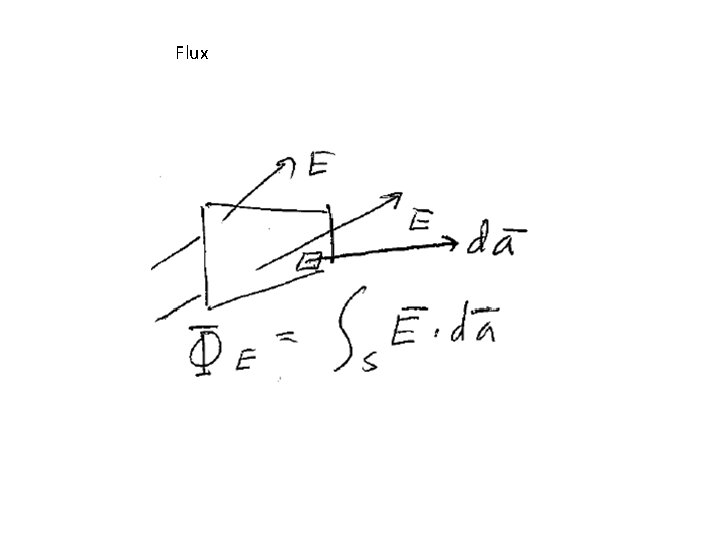FluxFlux through closed surface is proportional to the amount of charge inside Field lines Closed surfaceDifferent closed surface No net flux: All field lines that enter from the left, exit on the right.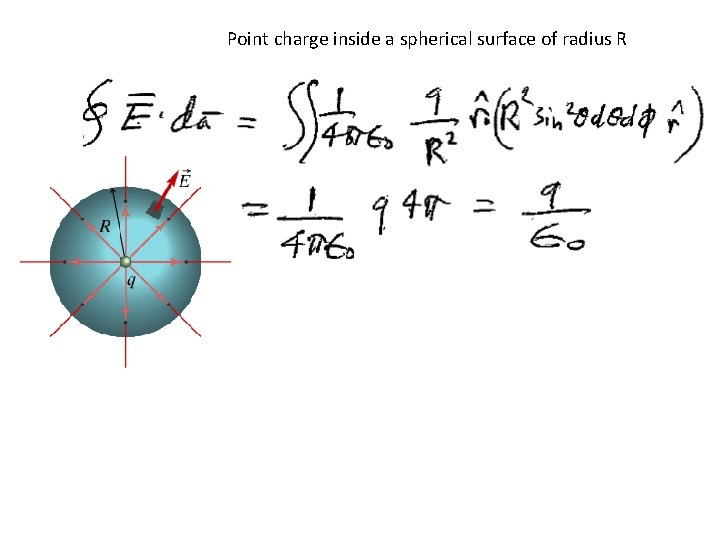Point charge inside a spherical surface of radius RMultiple point charges Gauss’s Law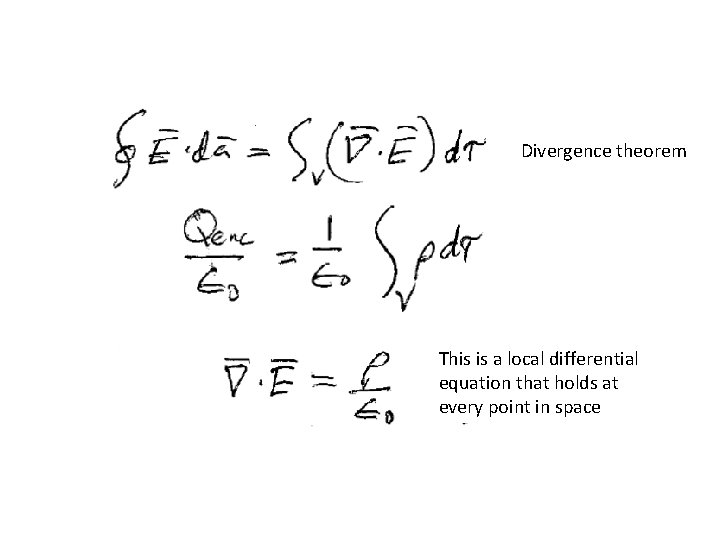Divergence theorem This is a local differential equation that holds at every point in space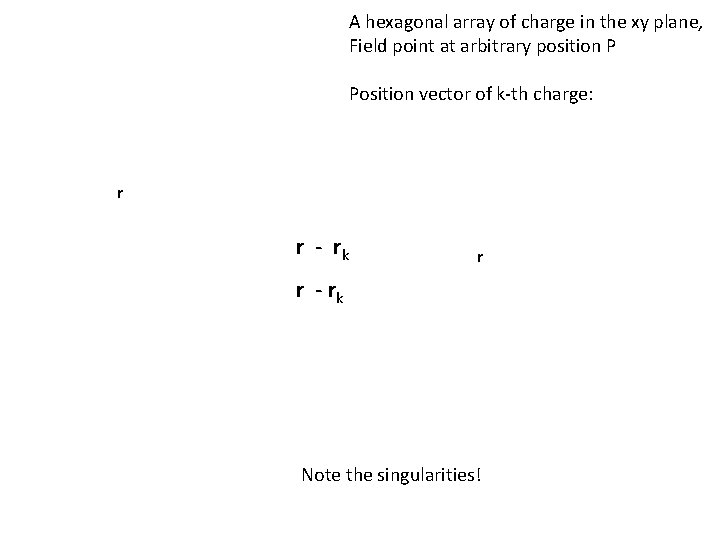A hexagonal array of charge in the xy plane, Field point at arbitrary position P Position vector of k-th charge: r r - rk Note the singularities!The sum over the y components of this vector will be zero. What is the electric field if field point P is on the x-axis? q Denominator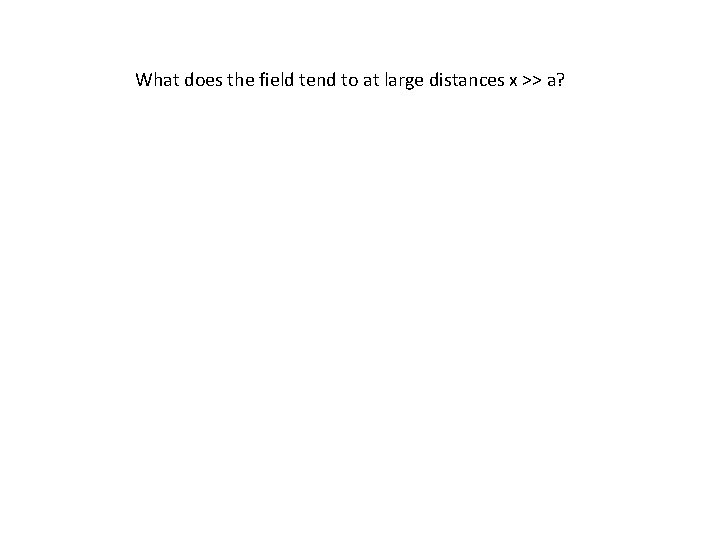What does the field tend to at large distances x >> a?Large distances At large distances, the charge distribution appears to collapse to a point, so field is that of a point charge. For higher precision do a Taylor expansion: The Multipole Expansion!What is the field at the origin, i. e. at the center of this symmetrical charge distribution? What if we remove one of the charges, how do we find the field at the origin now?Remove charge 6 from the group. That is the same as adding an equal an opposite charge at that position to “cancel” the original charge.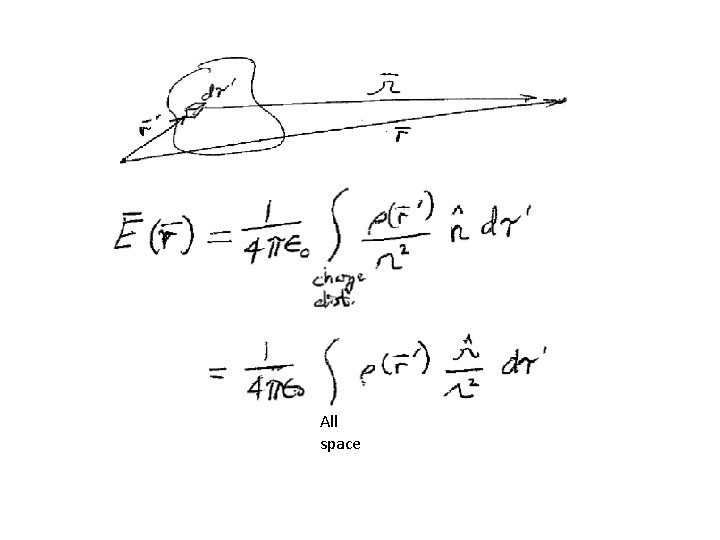All space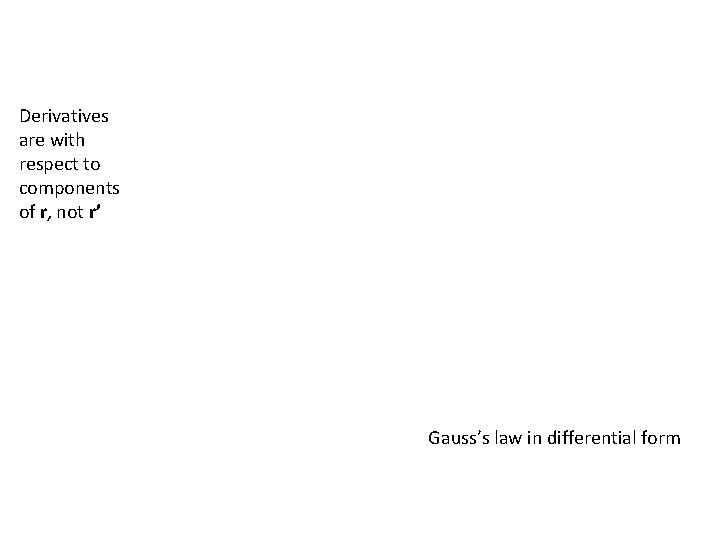Derivatives are with respect to components of r, not r’ Gauss’s law in differential form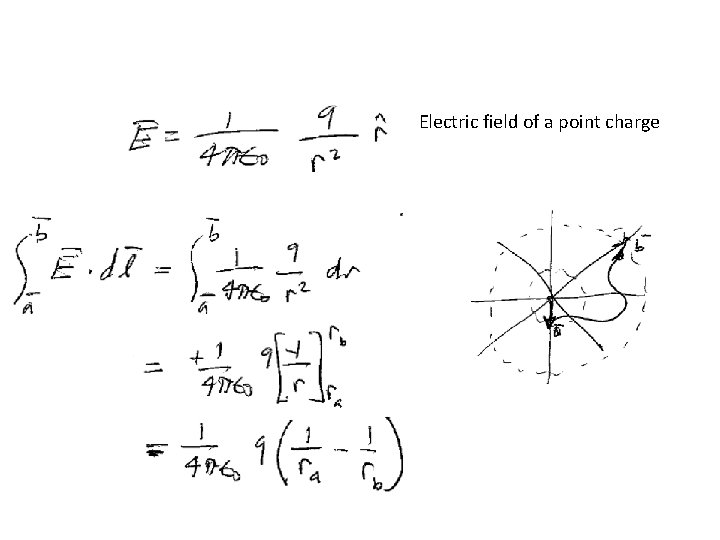Electric field of a point charge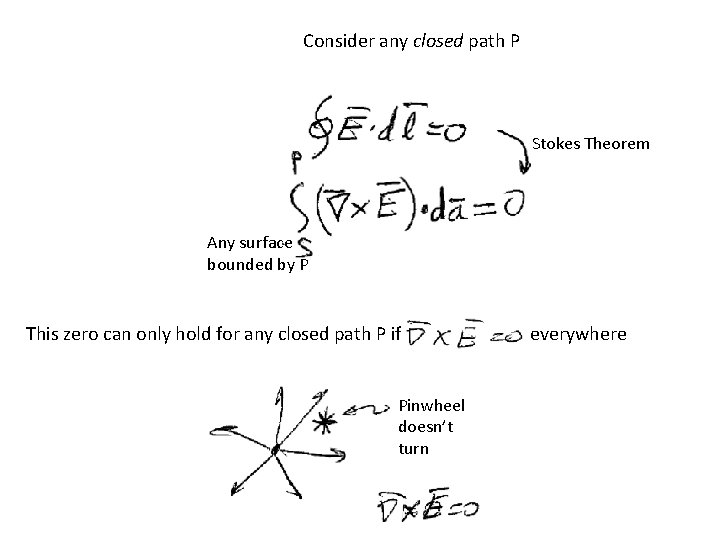Consider any closed path P Stokes Theorem Any surface bounded by P This zero can only hold for any closed path P if Pinwheel doesn’t turn everywhere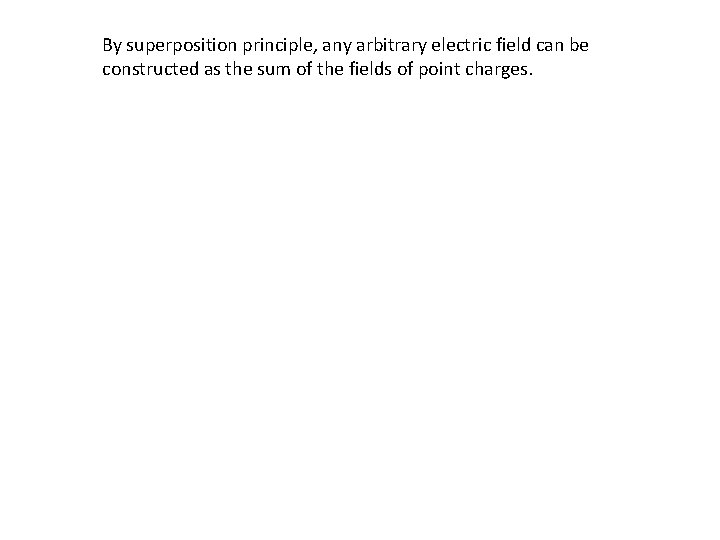By superposition principle, any arbitrary electric field can be constructed as the sum of the fields of point charges.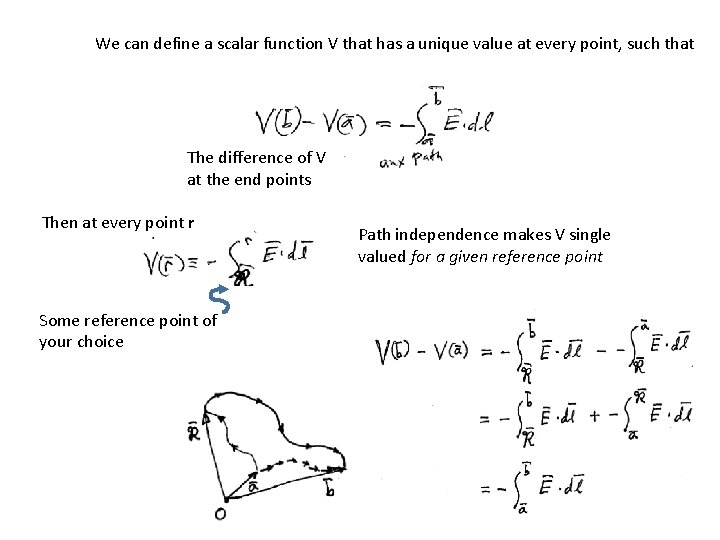We can define a scalar function V that has a unique value at every point, such that The difference of V at the end points Then at every point r Some reference point of your choice Path independence makes V single valued for a given reference point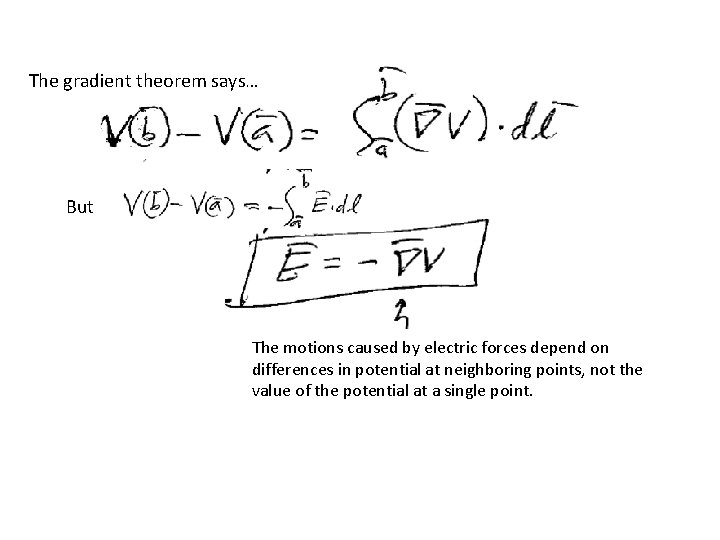The gradient theorem says… But The motions caused by electric forces depend on differences in potential at neighboring points, not the value of the potential at a single point.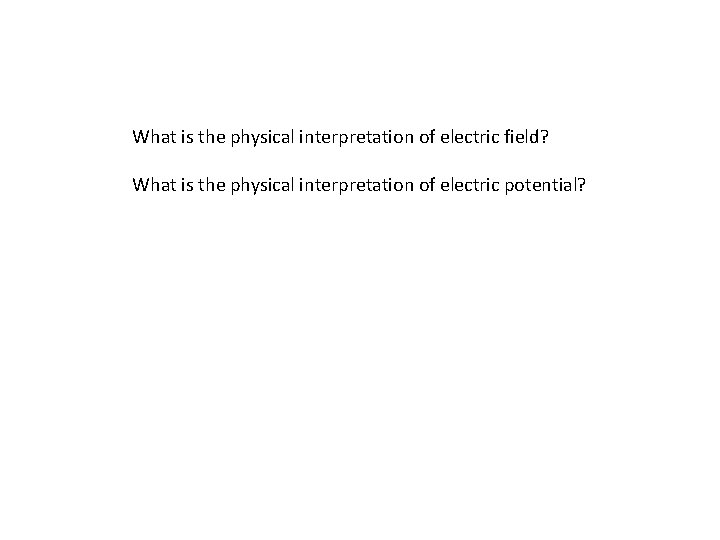What is the physical interpretation of electric field? What is the physical interpretation of electric potential?Superposition: The potential at a given point is the scalar sum of potentials from individual charges at that point. gives It is easier to work with scalar functions.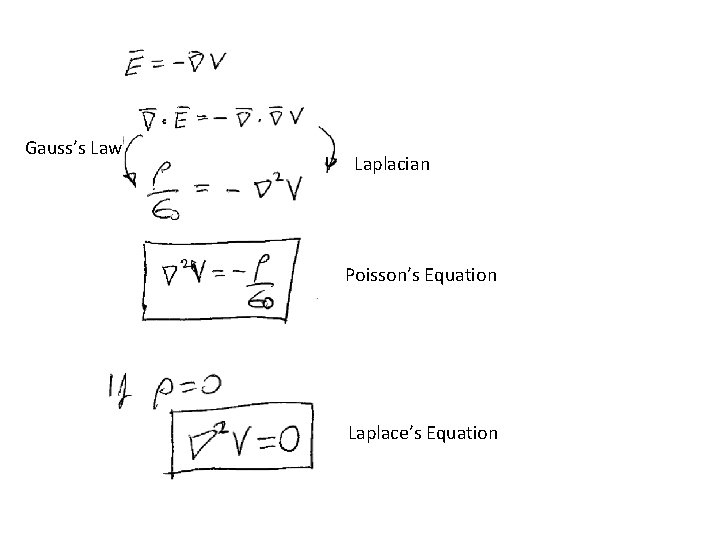Gauss’s Law Laplacian Poisson’s Equation Laplace’s Equation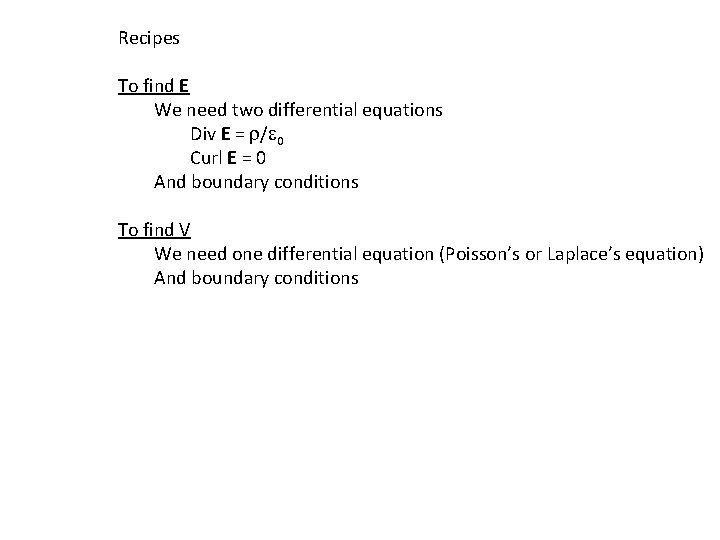Recipes To find E We need two differential equations Div E = r/e 0 Curl E = 0 And boundary conditions To find V We need one differential equation (Poisson’s or Laplace’s equation) And boundary conditions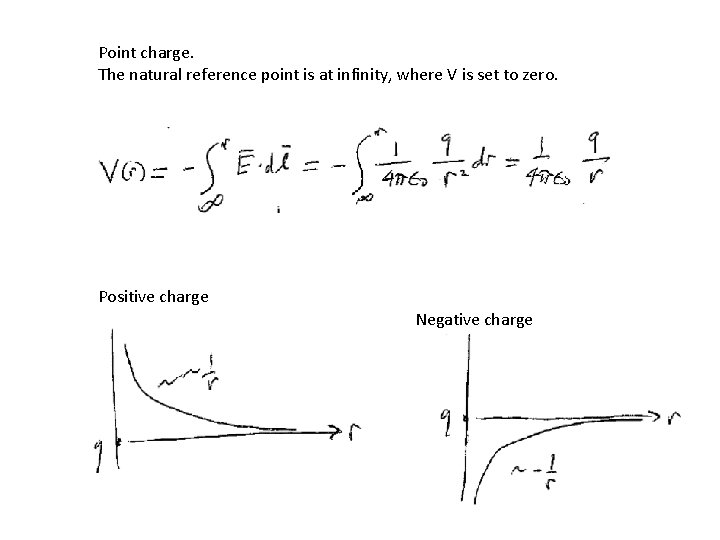Point charge. The natural reference point is at infinity, where V is set to zero. Positive charge Negative charge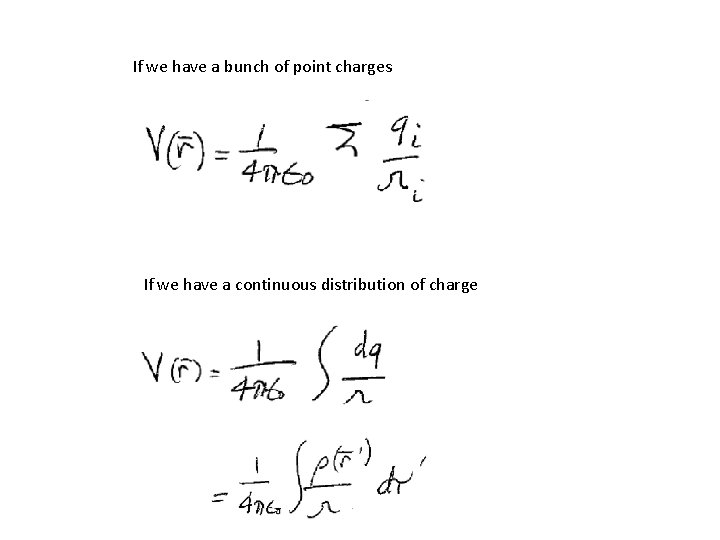If we have a bunch of point charges If we have a continuous distribution of charge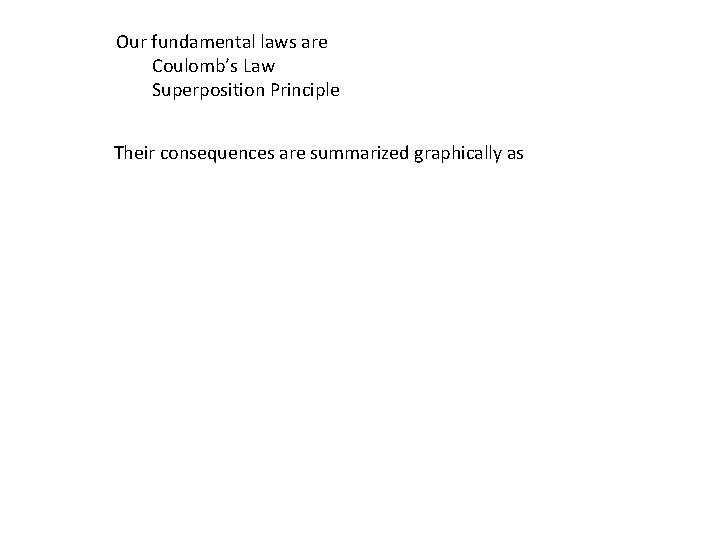Our fundamental laws are Coulomb’s Law Superposition Principle Their consequences are summarized graphically as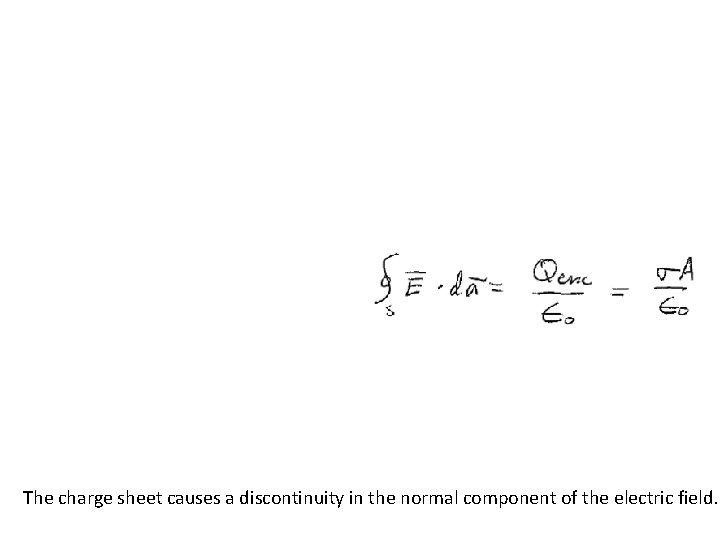The charge sheet causes a discontinuity in the normal component of the electric field.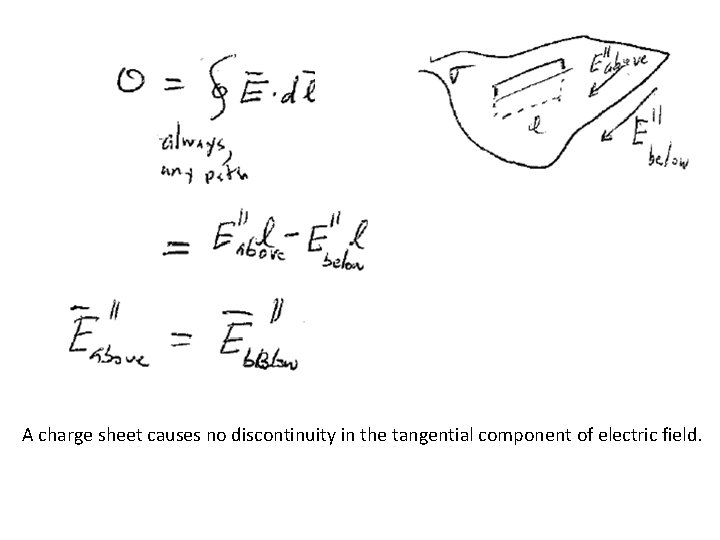A charge sheet causes no discontinuity in the tangential component of electric field.Combine the two boundary conditions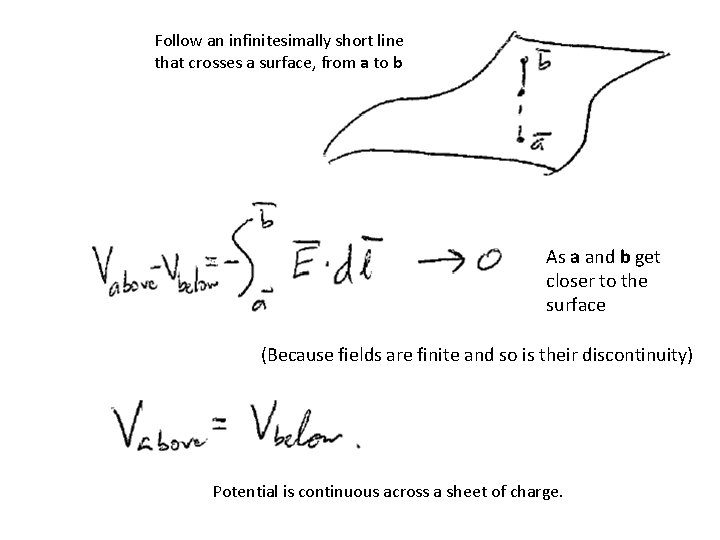Follow an infinitesimally short line that crosses a surface, from a to b As a and b get closer to the surface (Because fields are finite and so is their discontinuity) Potential is continuous across a sheet of charge.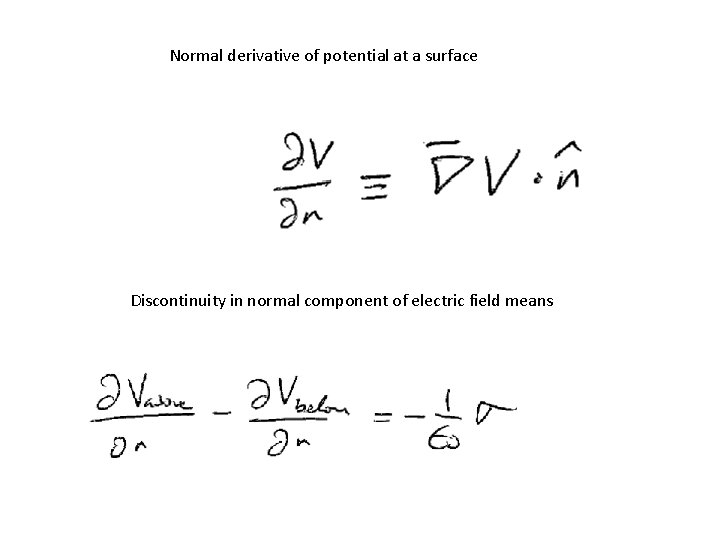Normal derivative of potential at a surface Discontinuity in normal component of electric field means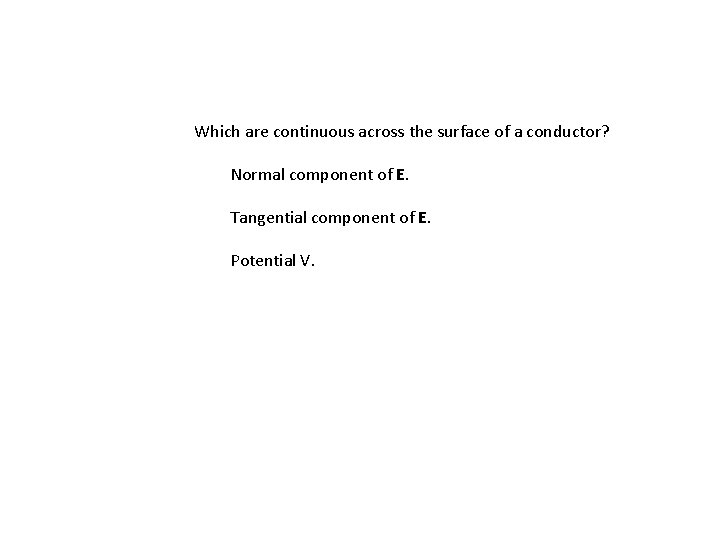Which are continuous across the surface of a conductor? Normal component of E. Tangential component of E. Potential V.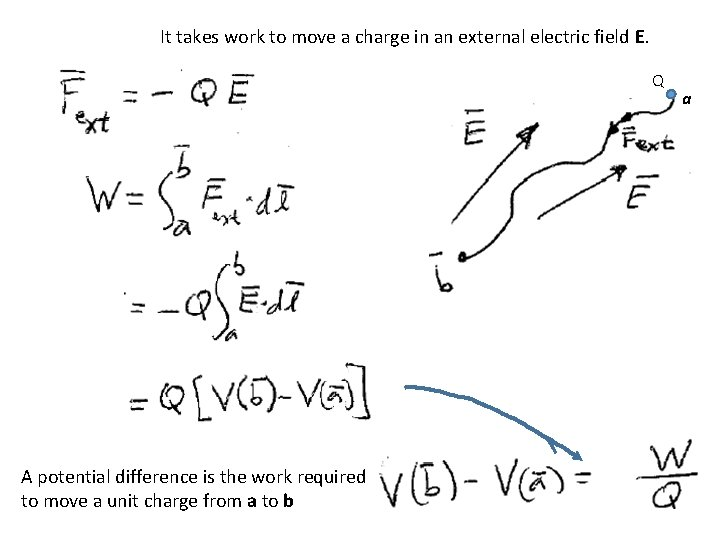It takes work to move a charge in an external electric field E. Q A potential difference is the work required to move a unit charge from a to b a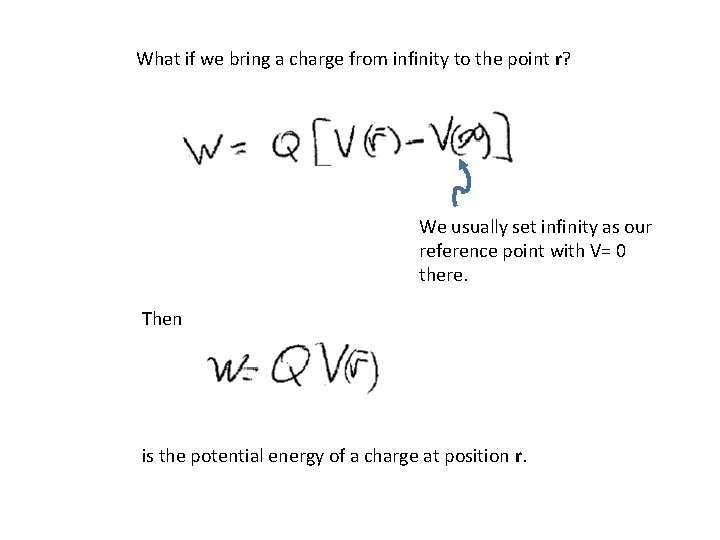What if we bring a charge from infinity to the point r? We usually set infinity as our reference point with V= 0 there. Then is the potential energy of a charge at position r.What if we assemble a bunch of charges by bringing them in from infinity, one at a time? The first charge costs nothing. The second charge costs energy due to the field of the first. The third charge is moved in the field of the first two. The total work to assemble three charges is the sum of the three parts, which can be positive or negative.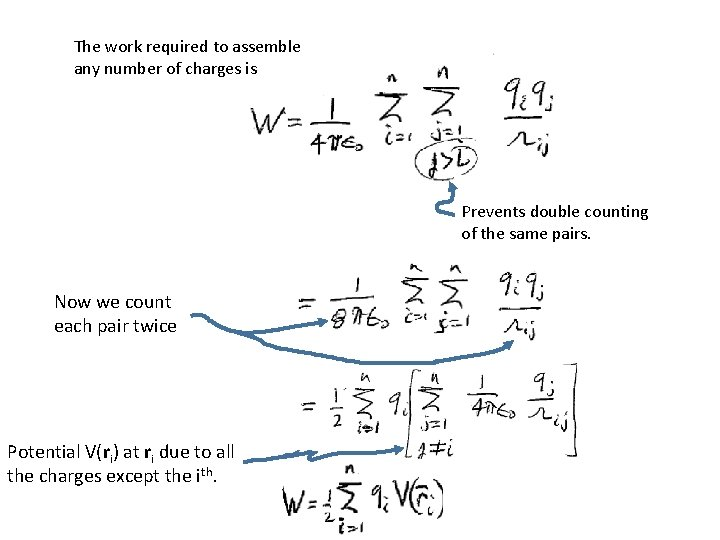The work required to assemble any number of charges is Prevents double counting of the same pairs. Now we count each pair twice Potential V(ri) at ri due to all the charges except the ith.What is the work required to assemble a continuous distribution of charge? Potential due to all the charge in the distribution including the amount within dt. (This will lead to a puzzle!) We can rewrite this same energy in terms of the electric field using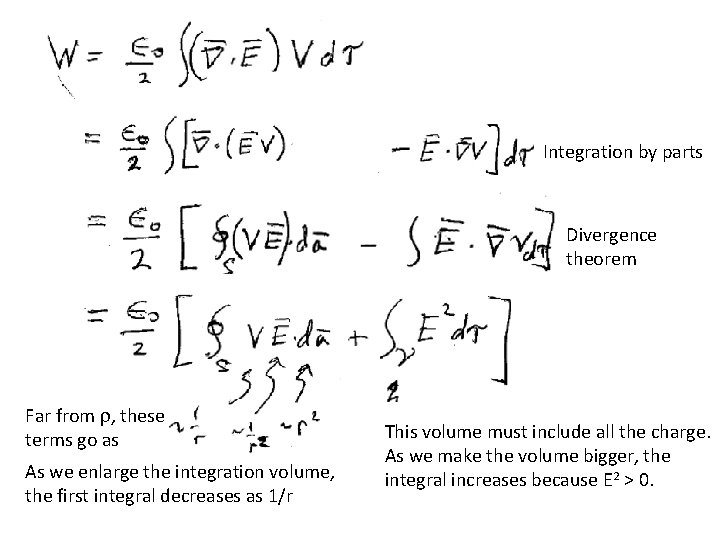Integration by parts Divergence theorem Far from r, these terms go as As we enlarge the integration volume, the first integral decreases as 1/r This volume must include all the charge. As we make the volume bigger, the integral increases because E 2 > 0.Enlarge the integration volume to include all space. The surface integral vanishes. All space The work required to assemble the charge distribution is stored as potential energy in the resulting electric field. This is always positive, but that wasn’t obvious from our first expression for W in terms of q and V. u= Field energy per unit volume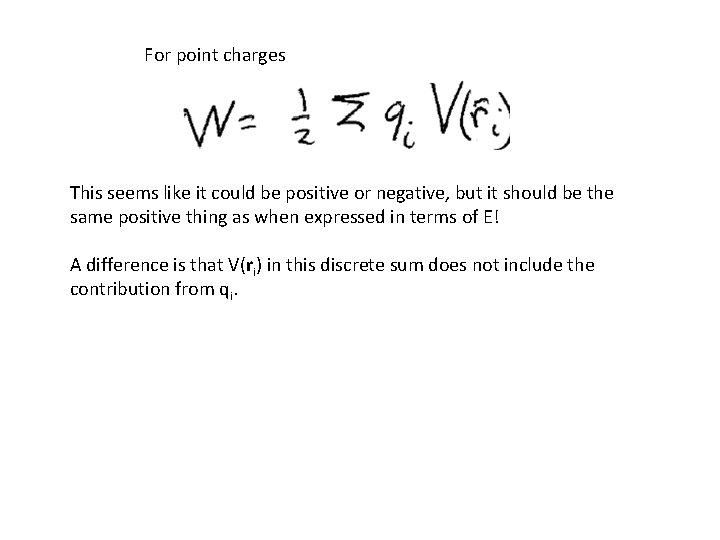For point charges This seems like it could be positive or negative, but it should be the same positive thing as when expressed in terms of E! A difference is that V(ri) in this discrete sum does not include the contribution from qi.Suppose we have two charge distributions, each giving rise to its own field at every point. The electric field obeys the superposition principle, but the energy W does not.The electrostatic electric field inside a conductor is zero. A non-zero field would cause current. Then there would be dissipation of energy. Then the state of the conductor would not be stationary. Turn on E 0 This is not a stationary state When an equilibrium stationary state is established, induced charges screen the interior, where the total field is E 0 + Ei = 0.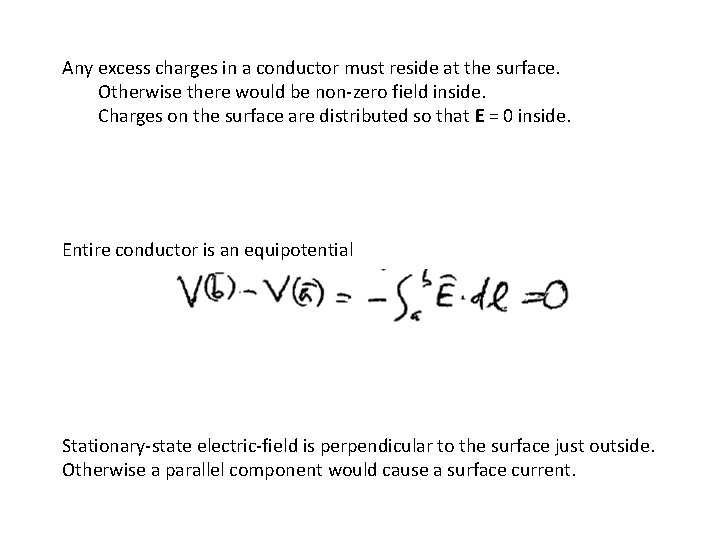Any excess charges in a conductor must reside at the surface. Otherwise there would be non-zero field inside. Charges on the surface are distributed so that E = 0 inside. Entire conductor is an equipotential Stationary-state electric-field is perpendicular to the surface just outside. Otherwise a parallel component would cause a surface current.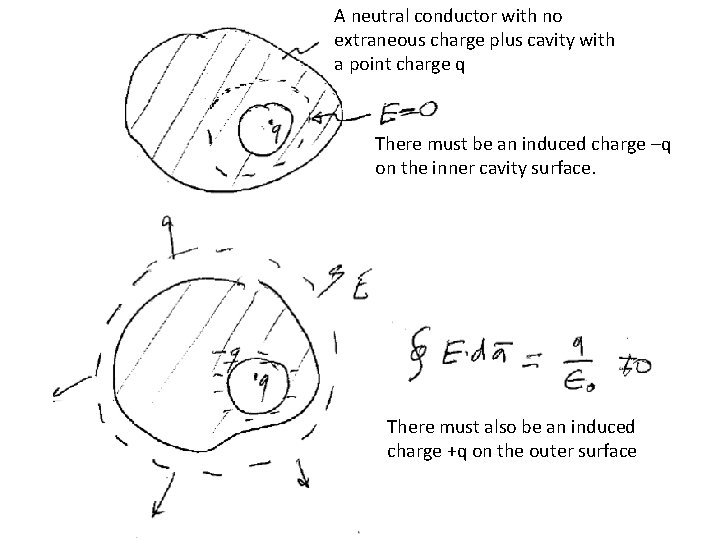A neutral conductor with no extraneous charge plus cavity with a point charge q There must be an induced charge –q on the inner cavity surface. There must also be an induced charge +q on the outer surface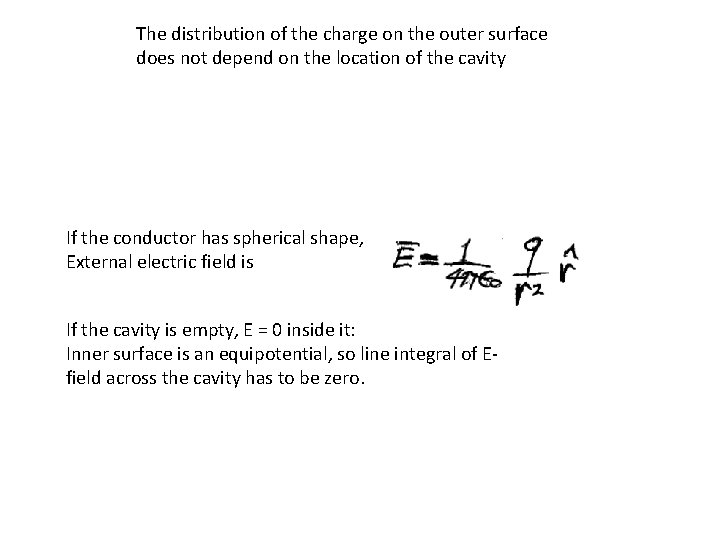The distribution of the charge on the outer surface does not depend on the location of the cavity If the conductor has spherical shape, External electric field is If the cavity is empty, E = 0 inside it: Inner surface is an equipotential, so line integral of Efield across the cavity has to be zero.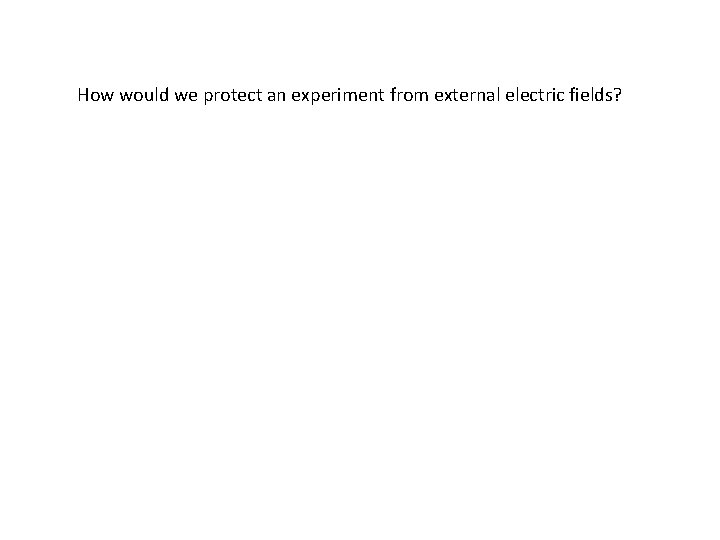How would we protect an experiment from external electric fields?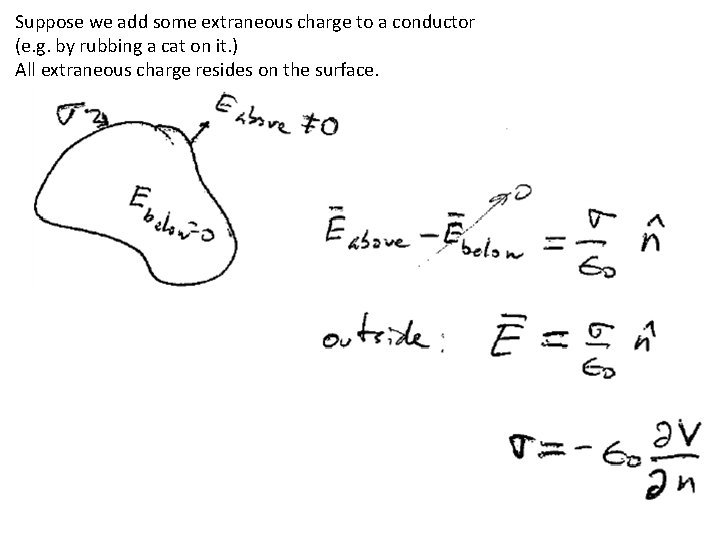Suppose we add some extraneous charge to a conductor (e. g. by rubbing a cat on it. ) All extraneous charge resides on the surface.The existence of an electric field on the outer surface of a conductor, but not on the inner, creates a force on the surface. The derivation of this force in chapter 2 is awkward. A simpler proof is based on the Maxwell stress tensor, which we will learn later. Force per unit area = This is a outward electrostatic pressure. Doesn’t depend on the sign of the charge.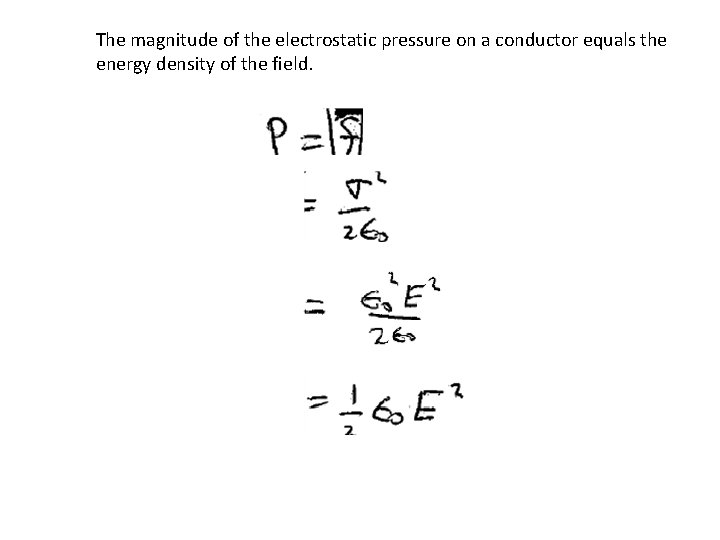The magnitude of the electrostatic pressure on a conductor equals the energy density of the field.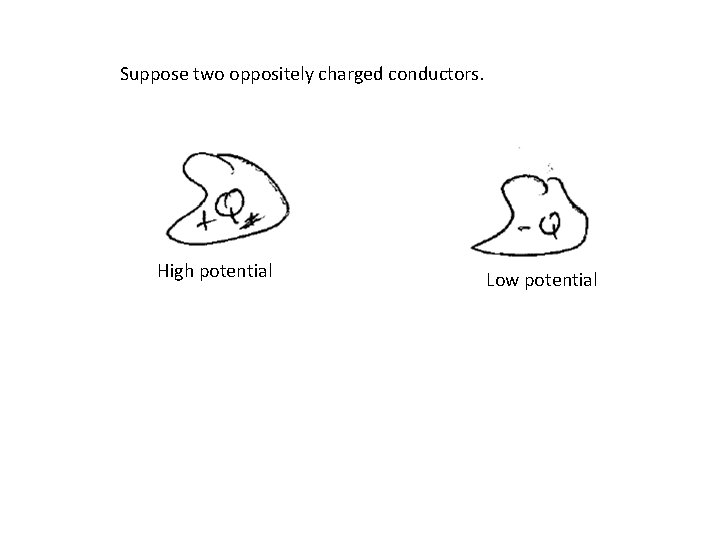Suppose two oppositely charged conductors. High potential Low potential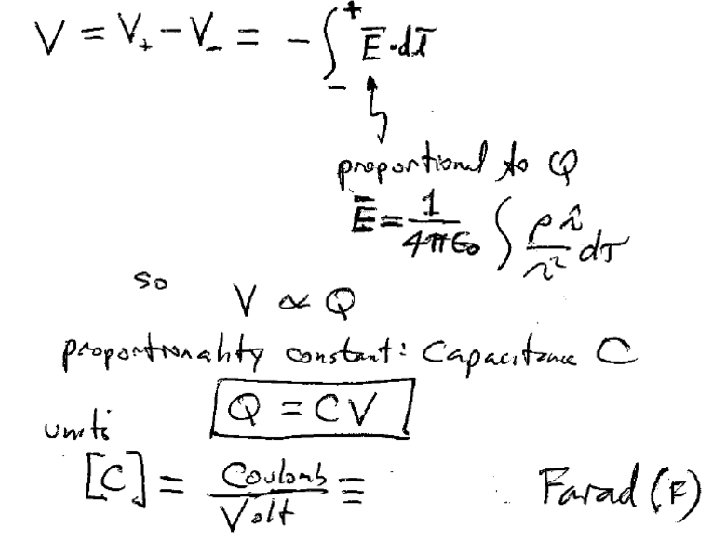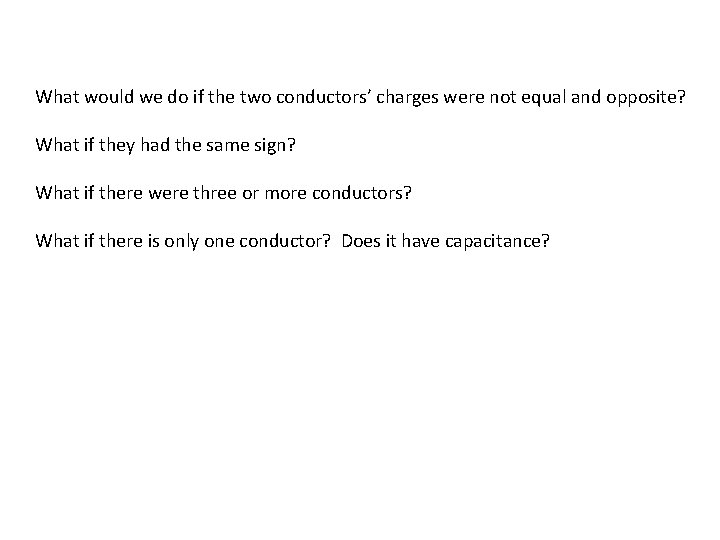What would we do if the two conductors’ charges were not equal and opposite? What if they had the same sign? What if there were three or more conductors? What if there is only one conductor? Does it have capacitance?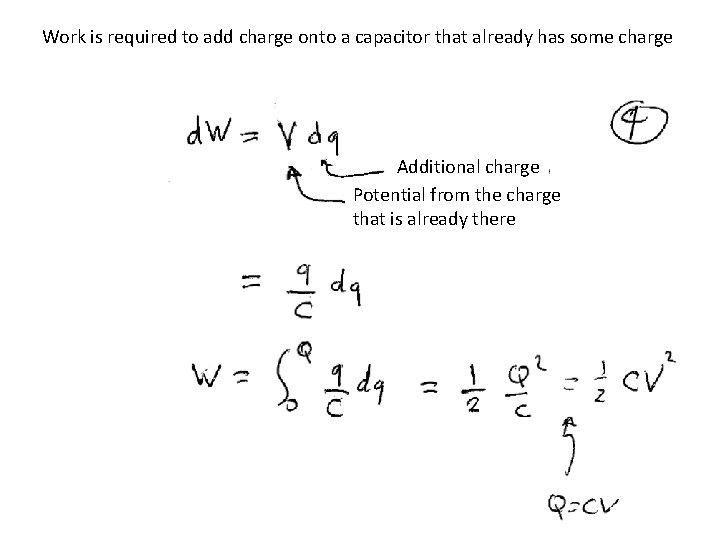Work is required to add charge onto a capacitor that already has some charge Additional charge Potential from the charge that is already there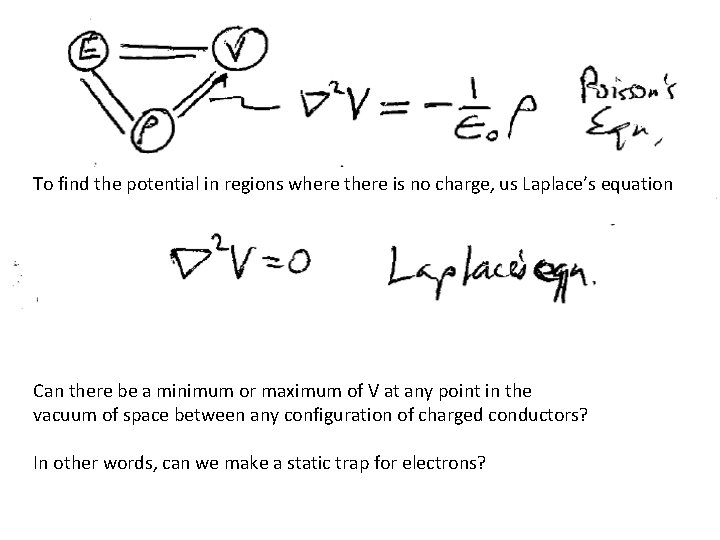To find the potential in regions where there is no charge, us Laplace’s equation Can there be a minimum or maximum of V at any point in the vacuum of space between any configuration of charged conductors? In other words, can we make a static trap for electrons?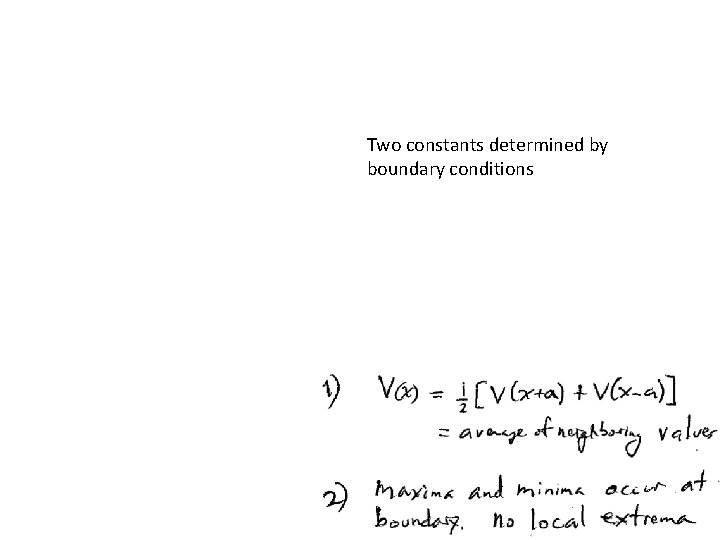Two constants determined by boundary conditions